JEE## Thermal Physics An Important Concept of JEE

The chapter Thermal Physics covers the most important concepts for JEE Advanced and other competitive exams. In the Thermal Physics chapter, students will study the basic heat transfer process and some laws related to the chapter.

The chapter on thermal physics begins with the very basic definition of Newton’s laws of cooling, the First law of thermodynamics and what are different other laws that are helpful in studying the chapter ahead. These concepts will lead us to a very important part of the chapter i.e. Reversible and Irreversible processes, Carnot engine, and efficiency. In this chapter, we will get to study many interesting and most important concepts such as thermal expansion, Specific heat capacity and Mean free path.

The concepts mentioned above will help us in studying the chapter  deeply along with the hands-on practice of many important numerical problems that will help us in acing the exams.

In this article, we will cover the important concepts and topics that will help students to revise and boost their preparations for JEE.

### Important Topics of Thermal Physics

• Heat Transfer

• First Law of Thermodynamics and Heat Capacity

• Second Law of Thermodynamics

• Newton’s Law of Cooling

• Reversible and Irreversible Processes

• Carnot Engine

• Temperature and Thermal Expansion

• Specific Heat Capacity and Mean Free Path

• Thermodynamic Variables

### Important Concepts of Thermal Physics

 Si.No Name of the Concept Key Points 1. Heat Transfer This process of heat transfer takes place in three formsConduction( this involves physical contact between two objects such that the heat transfer takes place from warmer body to cooler body)Convection( this takes place in the form of convection currents and it doesn’t require any physical touch between two bodies.)Radiation( this takes place in the form of rays that lie in the electromagnetic spectrum.) 2. Newton’s Law of Cooling This law states that the heat loss from anybody is directly proportional to the difference in temperature in the body and the corresponding surroundings.The formula for the law is given by$\dfrac{dT}{dt} = -k(T-T_{s})$ 3. Zeroth Law of Thermodynamics The law states that two systems in thermal equilibrium with a third system separately are in thermal equilibrium with each other. 4. First Law of Thermodynamics This law states that the energy supplied to the system goes in part to increase the internal energy of the system and rest in work done on the surroundings.The above statement can be formulated as-$\triangle Q$ = $\triangle U$ + $\triangle W$This law is in accordance with the Law of Conservation of Energy. 5. Heat Capacity Heat Capacity denoted by “ S” is defined as the minimum amount of heat required to raise the temperature of a substance from T to T + $\triangle T$This is a mass-dependent property.S = $\dfrac{\triangle Q}{\triangle T}$ 6. Specific Heat Capacity Specific heat capacity denoted by “s” is defined ass = $\dfrac{S}{m}$ or $\dfrac{\triangle Q}{m \triangle T}$This clearly shows that specific heat capacity is independent of mass and is a characteristic value of substance only. 7. Thermodynamic  State Variables These variables are of two types:-Extensive - These are those variables that are dependent on the mass of the system or the amount of quantity present in that system. E.g. Internal Energy, Volume and Mass.Intensive- These quantities are independent of the mass of the system like temperature, Pressure, Density. 8. Cyclic Process This process is defined as if a system returns to its initial state after performing a continuous cycle such that the change in internal energy is zero. 9. Heat Engine Heat engine is a device through which the cyclic process takes place in a system.It consists of a working substance which itself is a system.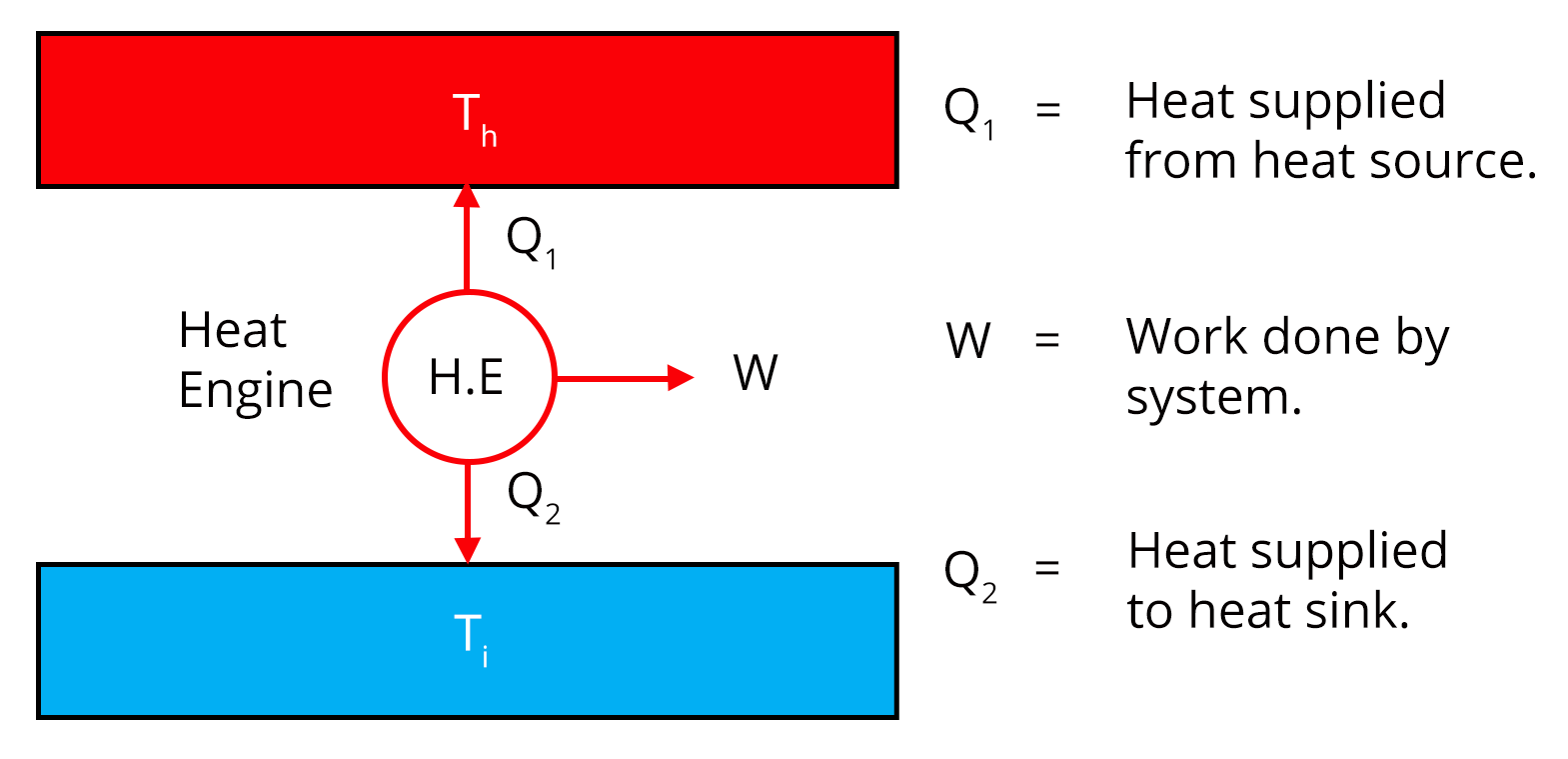If a heat engine has a source i.e. a hot reservoir having heat $Q_{1}$  at temp $T_{1}$ and a sink i.e. cold reservoir which releases heat $Q_{2}$ at temp $T_{2}$  then its efficiency is defined as$\eta = \dfrac{W}{Q_{1}}$Where W is the work done by the system in a cyclic process. 10. Second Law of Thermodynamics The law states that heat can’t be transferred from a body at a lower temperature to a body at a higher temperature without any addition of energy. 11. Reversible and Irreversible processes A thermodynamic process can be said to be reversible if the process returns to its original position from the initial i.e. both system and surroundings return to their original states without any change in the universe.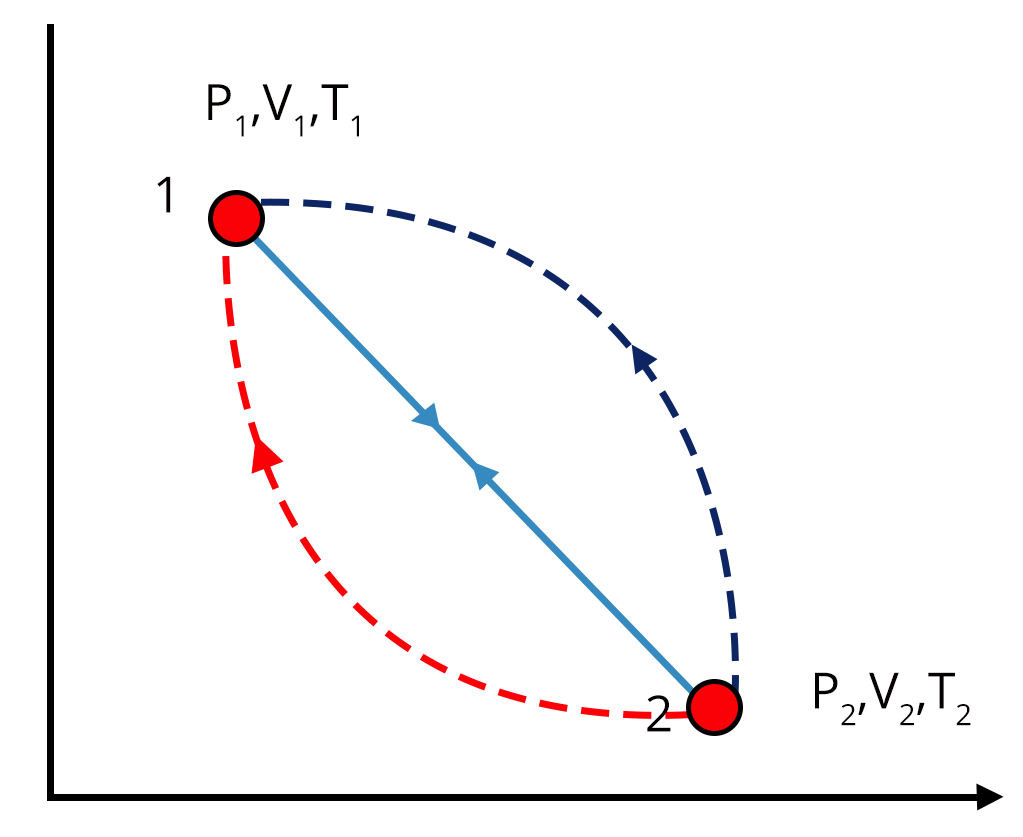Irreversible Processes are those that cannot return to their original conditions with the same path if reversed.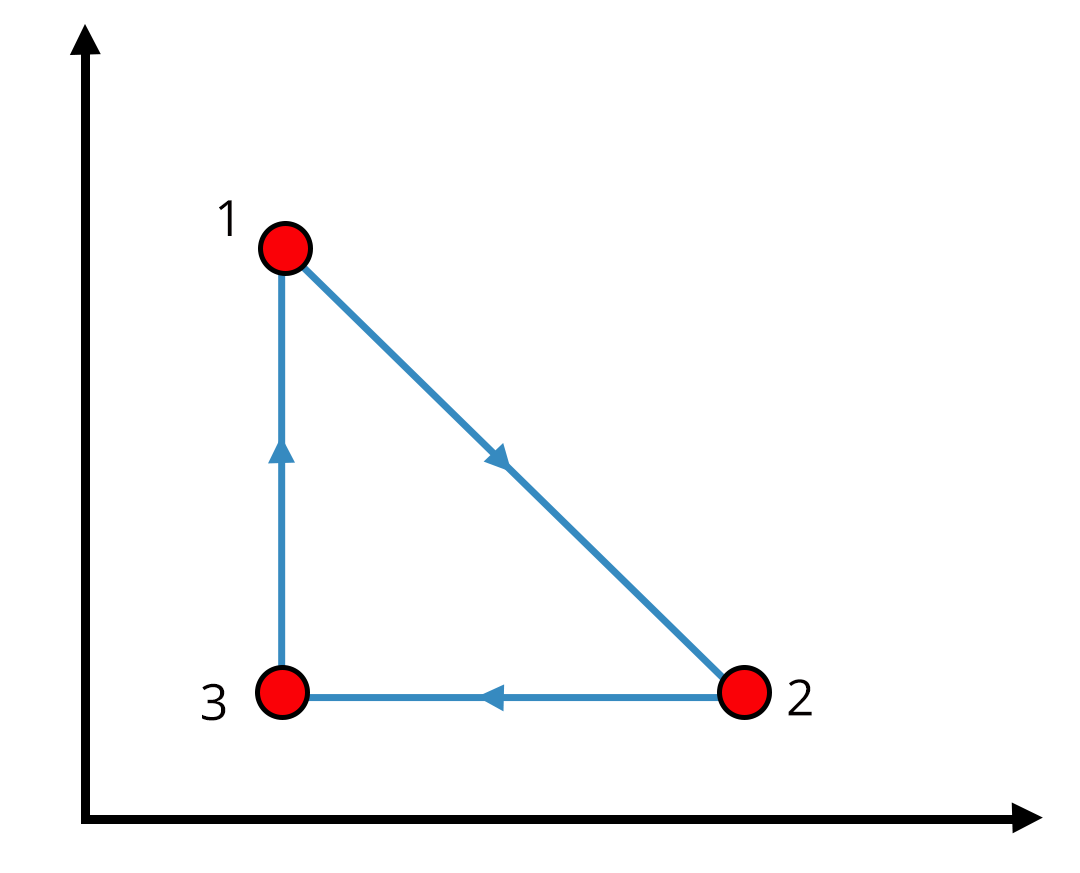12. Carnot Engine The Carnot engine is the only possible reversible that works between two reservoirs at different temperatures. Efficiency of Carnot engine is given as$\eta = 1-\dfrac{T_{2}}{T_{1}}$Another major formula used in Carnot Cycle which shows the interdependence of system is$\dfrac{T_{1}}{T_{2}}$ = $\dfrac{Q_{1}}{Q_{2}}$ 13. Processes in Carnot Cycle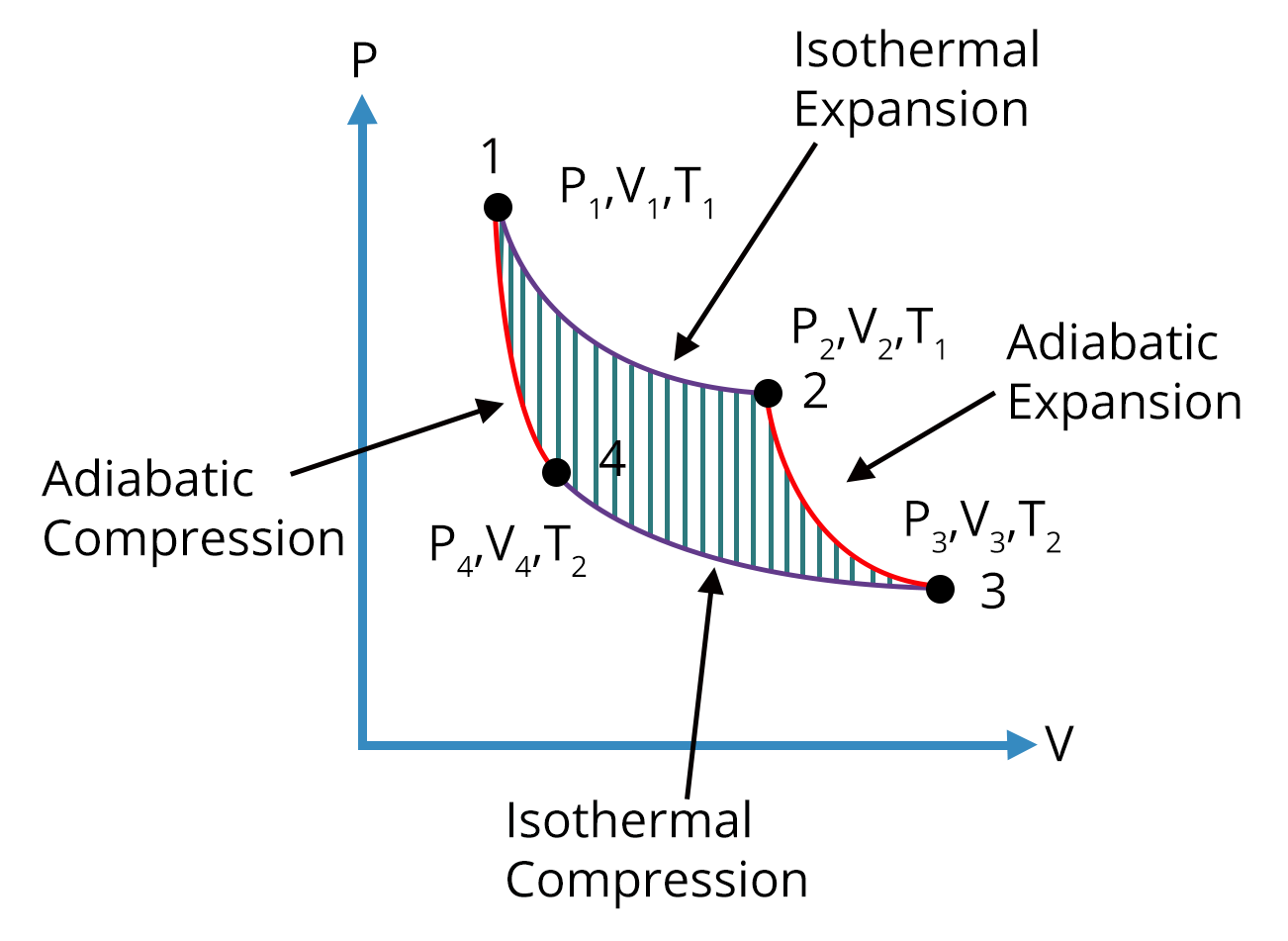Process 1-2 is Isothermal Expansion taking place at a constant temperature.Process 2-3 is Adiabatic Expansion such that no heat is absorbed or lost by the system.Process 3-4 is Isothermal Compression where heat is rejected by the system.Process 4-1 is Adiabatic Compression. 14. Distribution of Molecular Speeds For a given mass of gas the velocities of all molecules are not same even their macroscopic parameters are fixed hence Maxwell introduced various speeds likeMost probable speed-The speed which is attained by large no of molecules having value $v_{p} = 1.41\sqrt{\frac{kT}{m} }$Average speed- Average sum of all the speeds of molecules with value $v_{a} = 1.59\sqrt{\frac{kT}{m} }$Root mean squared - Value of this speed is $v_{rms} = 1.73\sqrt{\frac{kT}{M} }$ 15. Thermal Expansion A phenomenon in which substances expand on heating and contract on cooling with a change in their dimension is called Thermal Expansion. Depending on the shape of the body expansion is of three typesLinear ExpansionArea ExpansionVolume Expansion

### Different Thermodynamic Processes

 Isochoric Process Isothermal Process Adiabatic Process Isobaric Process This process takes place at const volume. That process in which the temperature of the system is kept constant is called Isothermal process. This process doesn’t allow any exchange of heat i.e. the system is an insulated one with zero amount of heat absorbed or released. That process in which pressure of the system is kept constant is called Isobaric process. For this process the work done in or by the system is zero.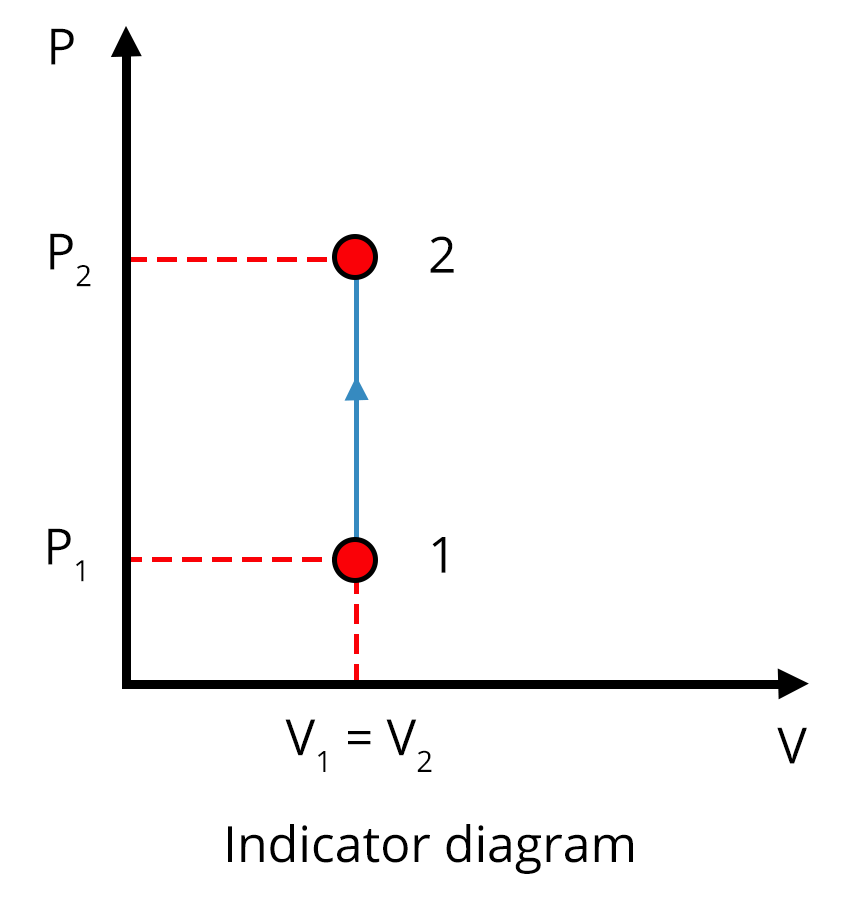Work done for this system is given by$nRT\times 2.303\times\log{\dfrac{V_{2}}{V_{1}}}$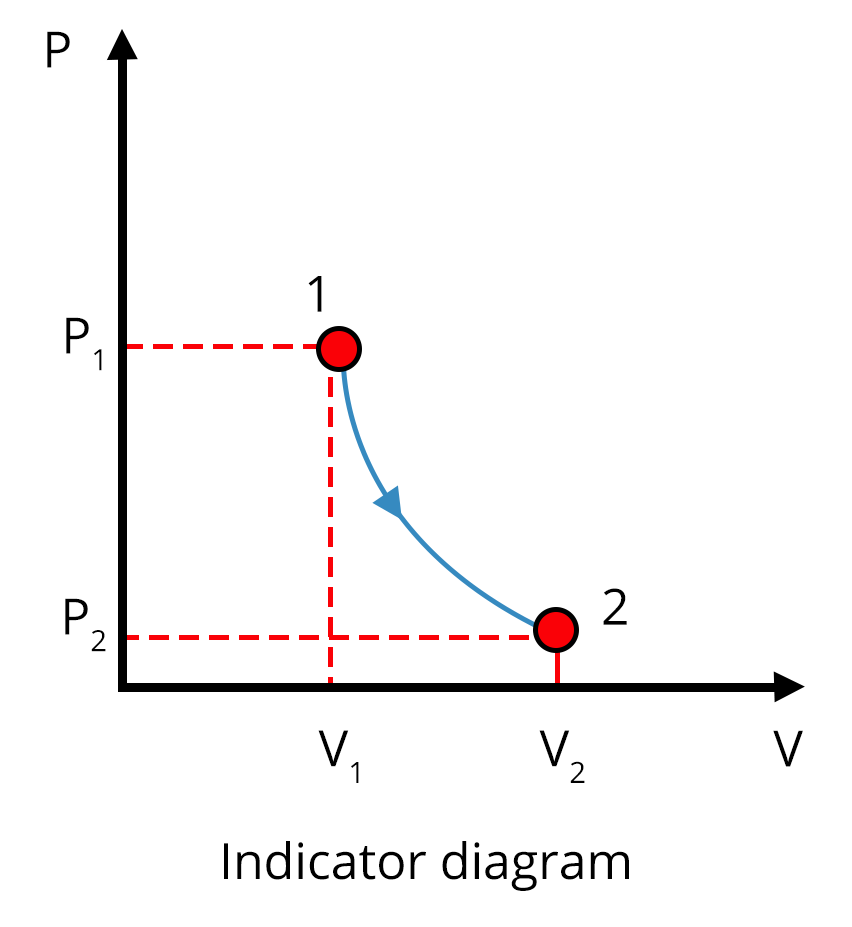Work done for this system is given by$\dfrac{nR(T_{1} - T_{2})}{\gamma -1}$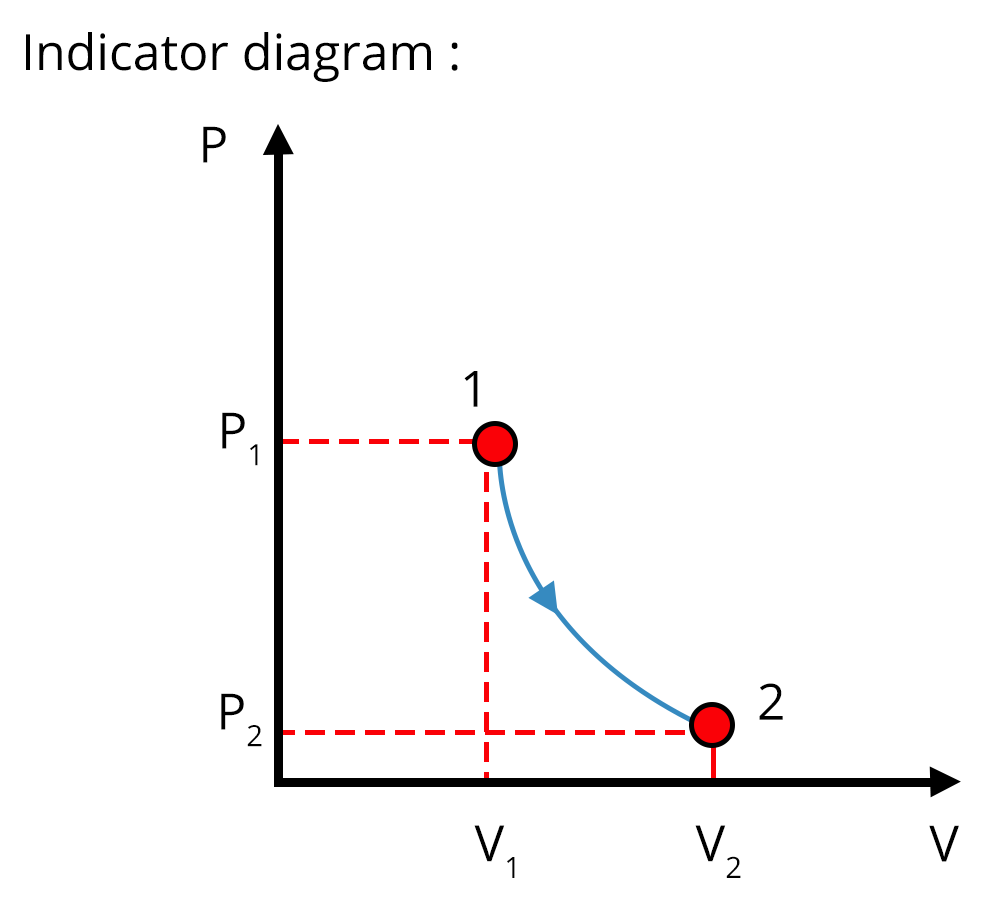Work done by the system for this process is W =nR$(T_{2} - T_{1})$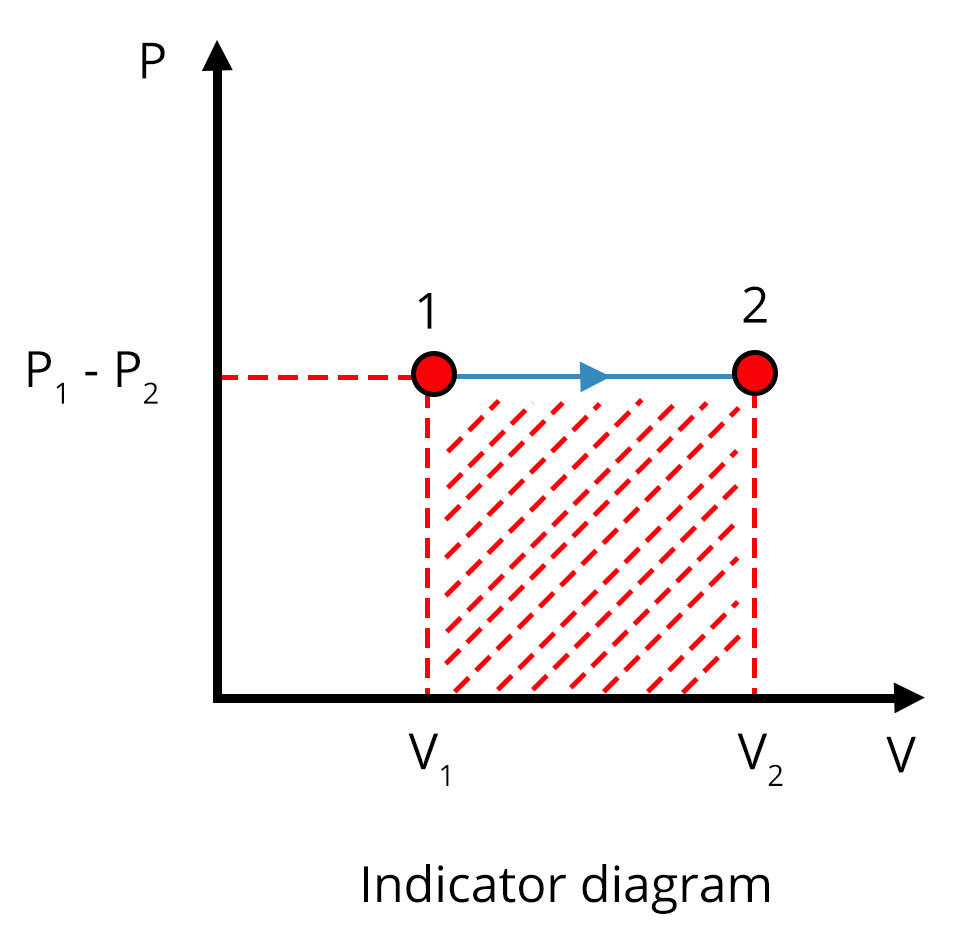### List of Important Formulae of Thermal Physics

 Sl. No Name of the Concept Formulae 1. Newton’s Law of Cooling $\dfrac{dT}{dt} = -k(T-T_{s})$ 2. First Law of Thermodynamics $\triangle Q$ = $\triangle U$ + $\triangle W$ 3. Heat Capacity S = $\dfrac{\triangle Q}{\triangle T}$ 4. Specific Heat Capacity s = $\dfrac{S}{m}$ or $\dfrac{\triangle Q}{m \triangle T}$ 5. Efficiency of heat engine $\eta = \dfrac{W}{Q_{1}}$ 6. Coefficient of Performance $\alpha = \frac{1-\eta}{\eta}$ 7. Work done by Isochoric Process For this process the work done in or by the system is zero. 8. Work done by Isothermal Process $nRT\times 2.303\times\log{\dfrac{V_{2}}{V_{1}}}$ 9. Work done by Isobaric Process W =$nR(T_{2} - T_{1})$ 10. Work done by Adiabatic Process $\dfrac{nR(T_{1} - T_{2})}{\gamma -1}$ 11. Carnot Engine’s Efficiency $\eta = 1-\dfrac{T_{2}}{T_{1}}$ 12. Most probable speed $v_{p} = 1.41\sqrt{\frac{kT}{m} }$ 13. Average speed $v_{a} = 1.59\sqrt{\frac{kT}{m} }$ 14. Root mean squared $v_{rms} = 1.73\sqrt{\frac{kT}{M} }$ 15. Mean free path $\lambda= \dfrac{1}{\sqrt{2}n\pi d^{2}}$ 16. Linear Expansion $\dfrac{\Delta l}{l}= \alpha_{l}\Delta T$

### Solved Examples of Thermal Physics

1. A steam engine delivers 5.4 x 108 J of work per minute and services 3.6 x 109 J of heat per minute from its boiler. What is the efficiency of the engine? How much heat is wasted per minute?

Sol:

Given,

Work done by the boiler per minute (output work) = 5.4 x 108 J

Input work heat absorbed by boiler = 3.6 x 109 J

Hence efficiency = $\eta = \dfrac{\text{output}}{\text{input}}$

= $\dfrac{5.4\times 108} {3.6\times 109}$

=0.15

So the heat wasted per minute is equal to the difference between heat absorbed per minute and output work per minute.

= $\left(3.6 \times {10^9}\right)- \left (5.4 \times {10^8}\right) J$

=$3.06 \times {10^9} J$

Therefore, the efficiency of the engine is 0.15 and heat is wasted per minute.

Key Point: The relationship between the output work and input work is given by relation $\eta = \dfrac{\text{output}}{\text{input}}$

2. A cylinder with a movable piston contains 3 moles of hydrogen at standard temperature and pressure. The walls of the cylinder are made of a heat insulator, and the piston is insulated by having a pile of sand on it. By what factor does the pressure of the gas increase if the gas is compressed to half its original volume?

Sol:

Given,

The cylinder is undergoing adiabatic compression hence for a diatomic gas(hydrogen)  the value of $\gamma$ = 1.4 and as mentioned in the ques final volume = half of the initial one

$V_{2} = \frac{V_{1}}{2}$

$P_{2}$ = 1atm

$P_{1}V_1^\gamma$ = $P_{2}V_2^\gamma$

$V_1^\gamma$ = $P_{2}V_2^\gamma$

$V_1^\gamma$ = $P_{2}(\frac{V_{1}}{2})^{\gamma}$

Hence $P_{2}= 2^{1.4}$

=2.64atm

Therefore, 2.64 atm pressure of the gas increase if the gas is compressed to half its original volume.

Key point: In the case of adiabatic processes, the system is always an insulated one so that no heat can be absorbed or released by the system.

### Previous Year Questions of Thermal Physics

1. Under isothermal conditions, a gas at 300 K expands from 0.1 L to 0.25 L against a constant external pressure of 2 bar . The work done by the gas is( Given that 1L bar=100J)(NEET 2019)

1. 5 kJ

2. 25 J

3. 30 J

4. -30 J

Sol:

Given,

Pressure of the gas = 2bar

Change in volume = $\triangle V$ = 0.25-0.1 = 0.15 L

Since the process is isothermal conditions hence temperature of gas = 0K

From 1st law of thermodynamics

$\triangle Q$ = $\triangle U$ + $\triangle W$

$\triangle Q$  = 0 which means

$\triangle U$ = - $\triangle W$

So the work done is calculated as $\triangle W$ = - $P\triangle V$

=-2$\times$ 0.15 = -0.3L-bar

And as given in ques 1L-bar = 100 J

Hence net work done in Joule is -30J Which is option (d)

Trick: As the given process is isothermal and by the formula of thermodynamics one can see that work done will be negative. So without calculations just by looking at the options, a student can go for an option with a negative sign.

2. A Carnot freezer takes heat from water at $0^{\circ} C$ inside it and rejects it to the room at a temperature of $27^{\circ}C$. The latent heat of ice is $336\times10^{3}$ J/kg. If 5 kg of water at $0^{\circ}C$ is converted into ice at $0^{\circ}C$ by the freezer, then the energy consumed by the freezer is close to:  (JEE 2016)

1. $1.67\times10^{5}$ J

2. $1.68 \times10^{6}$ J

3. $1.51\times10^{5}$ J

4. $1.71 \times10^{7}$ J

Sol:

Given,

Temperature of sink = $T_{sink}$ = $0^{\circ} C$ = 273K

Temperature of source = $T_{source}$ = $27^{\circ} C$ = 300K

Now the latent energy required by freezer to convert ice to water will be the heat rejected by the freezer i.e. $Q_{sink}$ = mL

= 5 $\times 336 \times 10^{3}$ = $1680 \times 10^{3}$ J

From the formula of carnot engine

$\dfrac{T_{sink}}{T_{source}}$ = $\dfrac{Q_{sink}}{Q_{source}}$

Hence $\dfrac{273}{300}$ =$\dfrac{1680 \times 10^{3}}{Q_{source}}$

Or ${Q_{source}}$= $1846.1 \times 10^{3}$ J

So the energy consumed by the freezer = ${Q_{source}}$ - ${Q_{sink}}$

=$1846.1 \times 10^{3}$- $1680 \times 10^{3}$J

= $1.67\times10^{5}$ J which is option (a)

Trick: Here both the conversion of ice into water which is used as latent heat, formula of Carnot engine play an important role in solving the ques.

### Practice Questions

1. A quantity of air at normal temperature is compressed (a) slowly (b) suddenly to one-third of its volume. Find the rise in temperature, if any in each case $\gamma$ = 1.4 (Ans-(a) zero (b) 150.6$^{\circ}C$)

2. The water of one kg mass at 373 K is converted into steam at the same temperature and at atmospheric pressure. On boiling, 1 cc of water takes a volume of 1671 cc. Calculate the change in internal energy of the system taking the heat of vaporization to be 540 cal/g.(Ans- 499.84k cal)

### Conclusion

We conclude that the chapter ‘Thermal Physics’ has many important concepts that will help us in scoring better marks in NEET and JEE. In this article, we covered thermal expansion, Heat engines, Efficiency and all other important concepts from the chapter along with solved and previous year questions. Students can test their knowledge with the help of practice questions.

See MoreView All Dates
JEE Advanced 2022 exam date and revised schedule have been announced by the NTA. JEE Advanced 2022 will now be conducted on 28-August-2022, and the exam registration closes on 11-August-2022. You can check the complete schedule on our site. Furthermore, you can check JEE Advanced 2022 dates for application, admit card, exam, answer key, result, counselling, etc along with other relevant information.
See More
AugustRelease of Application form
07-Aug-2022Last date to fill the form
11-Aug-202223-Aug-202228-Aug-202203-Sep-202203-Sep-202211-Sep-2022Declaration of Result
11-Sep-2022
View All DatesApplication Form
Eligibility Criteria
Reservation Policy
IIT Bombay has announced the JEE Advanced 2022 application form release date on the official website https://jeeadv.ac.in/. JEE Advanced 2022 Application Form is available on the official website for online registration. Besides JEE Advanced 2022 application form release date, learn about the application process, steps to fill the form, how to submit, exam date sheet etc online. Check our website for more details.View JEE Advanced Syllabus in Detail
It is crucial for the the engineering aspirants to know and download the JEE Advanced 2022 syllabus PDF for Maths, Physics and Chemistry. Check JEE Advanced 2022 syllabus here along with the best books and strategies to prepare for the entrance exam. Download the JEE Advanced 2022 syllabus consolidated as per the latest NTA guidelines from Vedantu for free.
See More
Paper 1
Paper 2MathsPhysics
View JEE Advanced Syllabus in Detail## JEE Advanced 2022 Study Material

View all study material for JEE Advanced
JEE Advanced 2022 Study Materials: Strengthen your fundamentals with exhaustive JEE Advanced Study Materials. It covers the entire JEE Advanced syllabus, DPP, PYP with ample objective and subjective solved problems. Free download of JEE Advanced study material for Physics, Chemistry and Maths are available on our website so that students can gear up their preparation for JEE Advanced exam 2022 with Vedantu right on time.
See More
All
Chemistry
Maths
Physics
See Allsee all
See More
PYQP
Sample Paper
2021
All Years
Paper 1
24th July 2021 - physicsEnglish  •   Shift 1views
24th July 2021 - chemistryEnglish  •   Shift 1views
30th July 2021 - mathematicsEnglish  •   Shift 1views
Paper 2
30th July 2021 - physicsEnglish  •   Shift 2views
04th August 2021 - chemistryEnglish  •   Shift 2views
04th August 2021 - mathematicsEnglish  •   Shift 2views
Paper 1
mathematicsEnglish  •   Shift NAviews
mathematicsEnglish  •   Shift NAviews
mathematicsEnglish  •   Shift NAviews
mathematicsEnglish  •   Shift NAviews
mathematicsEnglish  •   Shift NAviews
Paper 2
mathematicsEnglish  •   Shift NAviews
mathematicsEnglish  •   Shift NAviews
mathematicsEnglish  •   Shift NAviews
mathematicsEnglish  •   Shift NAviews
mathematicsEnglish  •   Shift NAviewsView all JEE Advanced Important Books
In order to prepare for JEE Advanced 2022, candidates should know the list of important books i.e. RD Sharma Solutions, NCERT Solutions, HC Verma books and RS Aggarwal Solutions. They will find the high quality readymade solutions of these books on Vedantu. These books will help them in order to prepare well for the JEE Advanced 2022 exam so that they can grab the top rank in the all India entrance exam.
See MoreMaths
NCERT Book for Class 12 MathsPhysics
NCERT Book for Class 12 Physics
See AllView all mock tests
See More
JEE Advanced 2018 Mock Test 1 - Paper 1
3 hr  • 69 questions • OBJECTIVE
JEE Advanced 2018 Mock Test 1 - Paper 2
3 hr  • 48 questions • OBJECTIVE
JEE Advanced 2018 Mock Test 2 - Paper 1
3 hr  • 69 questions • OBJECTIVE## Toppers## Master TeachersFrom IITs & other top-tier colleges with 5+ years of experienceYou can count on our specially-trained teachers to bring out the best in every student.They have taught over 4.5 crore hours to 10 lakh students in 1000+ cities in 57 countries11+ years exp

### Shreyas

Physics master teacher

#### NIT Nagpur4+ years exp

### Nidhi Sharma

Chemistry master teacher

#### Jamia Hamdard2+ years exp

### Luv Mehan

Chemistry Master Teacher

#### IISc BangaloreIIT Bombay is responsible for the release of the JEE Advanced 2022 cut off score. The qualifying percentile score might remain the same for different categories. According to the latest trends, the expected cut off mark for JEE Advanced 2022 is 50% for general category candidates, 45% for physically challenged candidates, and 40% for candidates from reserved categories. For the general category, JEE Advanced qualifying marks for 2021 ranged from 17.50%, while for OBC/SC/ST categories, they ranged from 15.75% for OBC, 8.75% for SC and 8.75% for ST category.
See MoreThe JEE Advanced 2022 result will be published by IIT Bombay on https://jeeadv.ac.in/ in the form of a scorecard. The scorecard will include the roll number, application number, candidate's personal details, and the percentile, marks, and rank of the candidate. Only those candidates who achieve the JEE Advanced cut-off will be considered qualified for the exam.
See More
Rank List
Counselling
Cutoff
JEE Advanced 2022 State Wise Rank List - The respective state authorities started releasing the JEE Advanced 2022 state merit lists for admission to Engineering course. Check JEE Advanced 2022 state-wise rank list for the year 2022 here.Want to know which Engineering colleges in India accept the JEE Advanced 2022 scores for admission to Engineering? Find the list of Engineering colleges accepting JEE Advanced scores in India, compiled by Vedantu. There are 1622 Colleges that are accepting JEE Advanced. Also find more details on Fees, Ranking, Admission, and Placement.
See More## FAQs on JEE Important Chapter - Thermal Physics

FAQ

1. Is Thermal Physics important for the JEE/NEET exam?

Yes, the thermal physics chapter is one of the important chapters for JEE and NEET exams. It carries around 2-3 questions. Due to the formula related questions from this chapter, it becomes easy to solve and secure marks in the exams.

2. Is the thermal physics chapter tough?

No, the thermal physics chapter is simple and easy to understand. The chapter includes the basic concepts that we got introduced to in our elementary school. If you understand the concepts discussed in this chapter and practice related questions then you can successfully attempt the questions asked in this chapter in both NEET and JEE exams.

3. Can I crack NEET by solving the previous years’ questions?

According to experts, applicants who practice previous year's papers for JEE and NEET have a good probability of passing the exam. Anyway, practice is the key to success. Students must focus on solving methods and study conceptually along with the PYQs.Last date for submitting JEE Advanced application form
12 Sep 202231 days remaining28 Sep 202246 days remaining## Notice board

News
BlogJEE Advanced 2022 Registration Ends Today
10 Aug 2022JEE Main 2022 Cutoff Released: Check Details Here
09 Aug 2022JEE Advanced 2022: Registration Window Open for Foreign Nationals
03 Aug 2022JEE Advanced 2022: Check Registration, Exam Pattern & Dates Details
29 Jul 2022JEE Main 2022 Results: Meet Vedans Who Scored 99.9 Percentile and Above
28 Jul 2022Trending pages